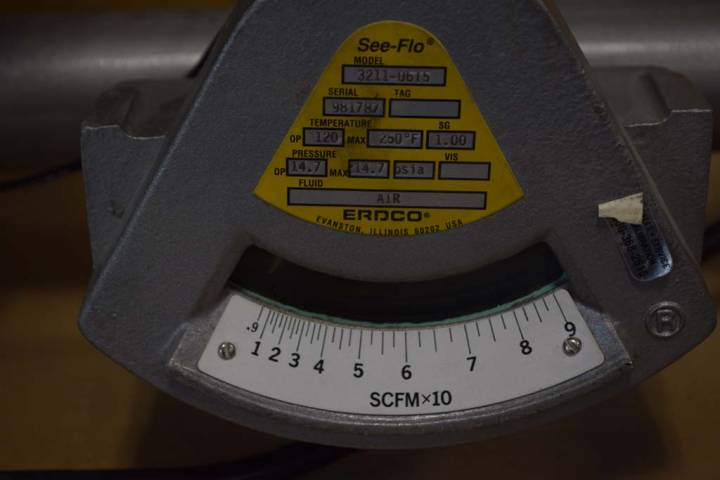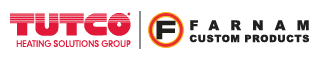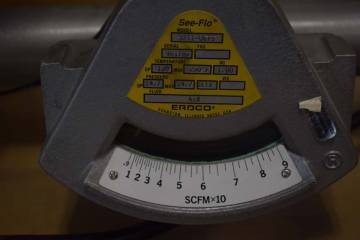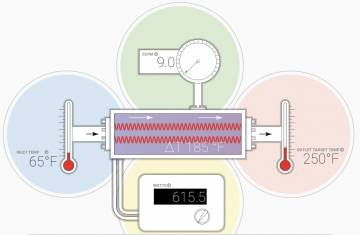Engineer Talk

# SCFM, CFM What’s the difference?## What’s the difference?

The majority of Tutco–Farnam Custom Product’s heaters are used to heat an air flow. When determining the power required to heat a stream of air, invariably two questions will come up.

1. How much “stuff” do we have to heat up? In more technical terms, what is the mass flow rate?
2. How hot does the air have to get? In other words, what is the temperature rise required?

We are going to concentrate on the first question. In the US, it is very common to see the volumetric air flow rate stated in units of Cubic Feet per Minute. Whether it is supplied by a fan, blower, or compressed air line, the volumetric air flow rate is simply stating the volume of air passing through over a given unit of time. SCFM is an acronym for Standard Cubic Feet per Minute, with “Standard” implying standard conditions. For the sake of this discussion, we are going to assume standard conditions of 1 atm and 68°F. Different industries and standard organizations actually cite slightly different values for what standard conditions are, but we are going to save that discussion for another time.

Cubic Feet per Minute = CFM = ft.3/minute at actual conditions

Standard Cubic Feet per Minute = SCFM = ft.3/minute at standard conditions (1 atm = 14.7psi, 68°F )

## Why do that?

A volumetric flow rate with units of CFM implies that the flow rate was measured at actual conditions (actual pressure, actual temperature). The distinction between SCFM and CFM is important because air density will change with pressure and temperature. This can be seen from taking a look at the ideal gas law. As you may remember, density = mass/volume. When the ideal gas law is rearranged, the air density can be seen to be proportional to pressure, and inversely proportional to temperature.

PV = mRT → ρ = m/V = P/RT

Air density at standard conditions = .0752 lb./ft3

Since the air density of an air flow stated at standard conditions (SCFM) is always the same, it is essentially a mass flow rate! Stating an air flow in units of SCFM makes it easy to compare conditions, and certain calculations simpler.

ρ = m/V → m = ρV →m/t = ρ (V/t)

mass flow rate = density* Volumetric flow rate

## How do I convert to SCFM?

We can easily derive the conversion equation from the ideal gas law and the mass flow rate equation above. The mass flow rate is equal whether the flow is stated in CFM or SCFM.

m/t = ρactual*CFM = ρstd*SCFM → SCFM = CFM *(ρactual/ ρstd) = CFM * (ρactual/Pstd)*(Tstd/Tactual)

Note: The ideal gas law uses absolute pressures and an absolute temperature scale.

SCFM = CFM * (Pactual/14.7psi)*(528°R/Tactual)

## Let’s put it to use

### Case 1

We need to heat up an air flow of 25 CFM at a pressure of 30 psig, and 90°F up to 200°F.

Convert flow to SCFM:

30 psig = 44.7 psia, 90°F = 550°R

SCFM = (25)*(44.7/14.7)*(528/550) = 73 SCFM

The equation Q/t = (m/t)*cp*ΔT can be approximated for air to:

Power [Watts] = (1/3)*SCFM*ΔT [°F]

Power [Watts] = (1/3)*73 SCFM* (200°F - 90°F) = 2676W

If we assume 15% more power for various losses, 3100W will be required.

### Case 2

We need to heat up an air flow of 200 CFM from 60°F up to 200°F using a blower operating at a static pressure of 2 in.H2O.

Convert flow to SCFM:

2 in.H2O = .072 psi = 14.772psia, 60°F = 520°R

SCFM = (200)*(14.772/14.7)*(528/520) = 204 SCFM

Power [Watts] = (1/3)*204 SCFM* (200°F - 60°F) = 9520W

If we assume 15% more power for various losses, 11kW will be required.

## Conclusion

For Case 1, if we had merely plugged in 25CFM into the above equation, we would have been undersized by a factor of about three. For Case 2, if we would have used 200CFM, we would have been under by 2% if we had not converted to SCFM.

Tutco-Farnam Custom Products### Power Flow Rate Interactive CalculatorThis interactive calculator helps explain the relationships between airflow, temperature and power. Click any of the circles to modify the inputs and automatically calculate the other factors. The graph at the bottom represents the ramp rate of outlet temperature over time given the parameters in the tool.

Calculators
Calculators +